# What are Musical Bar and Time Signature?

A Bar is a way to divide a song in equal time intervals, with the objective of organizing the structure and facilitating the orientation for the reader. This time interval is represented by vertical bars, as in the example below (highlighted in orange)

## Example of Musical Bar Lines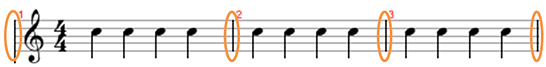In this example, what was the organization used for bars? It was to separate groups of 4 quarter notes. This means that 4 quarter notes can fit within each bar. This is the time interval defined for each bar, and there could be other figures in the middle, see: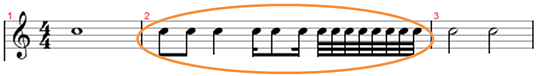In this example, pay attention to the second bar. There are several figures (quarter notes, eighth notes, sixteenth notes and thirty-second notes) in it, but all of them together occupy the length of 4 quarter notes, so they stay within the same bar. The same is true for bars 1 and 3, which have other figures equivalent to the length of 4 quarter notes.

Moral of the story: saying that “4 quarter notes can fit in one bar” does not mean that in one bar there can only be quarter note figures. This reference only tells us the time that a bar involves, regardless of the figures that are there.

Great, but what sets the time/length of a measure? Where is it written that each bar will have a length of 4 quarter notes?

Notice this fraction (time signature) below, which appears at the beginning of the sheet music we have just analyzed:

## Time Signature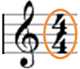This fraction 4/4 determined that a bar would have 4 quarter notes. Let’s find out why:

The fraction denominator: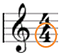Informs which figure will serve as a reference for the analysis. The number 4 refers to the quarter note, so this is the reference figure. The numerator: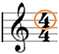Informs how many notes fit in each bar. Notice that the numerator of this fraction is saying that 4 notes can fit in a measure, and the denominator is saying that the note value is the quarter note, so the fraction 4/4 informs that 4 quarter notes can fit in one bar.

See the numbers that represent each note value in the denominator, in addition to the quarter note:

1 – Whole note

2 – Half note

4 – Quarter note

8 – Eighth note

16 – Sixteenth note

32 – Thirty-second note

64 – Sixty-fourth note

Let’s work on some examples of time signatures (take it as exercises) so that it becomes very clear how many notes fit in each measure:

4/4 = Fits 4 quarter notes

4/2 = Fits 4 half notes

4/8 = Fits 4 eighth notes

2/4 = Fits 2 quarter notes

3/1 = Fits 3 whole notes

5/32 = Fits 5 thirty-second notes

7/2 = Fits 7 half notes

## How to define a bar line in a song

The beat set for the bar is related to the pulse that the song has. Try to get some scores, listen to them and check their time signature. You will notice that the measure is marking: the rhythm of the song, the way the instrumentalists are dividing the chords or, simply, the rhythmic pattern that the melody follows.

Some musical styles generally already assume what their time will be. The waltz, for example, has a 3/4 time. In general, the most common time signature to appear in sheet music, undoubtedly, is 4/4. But there are countless possibilities for time signatures, so don’t just play “round” beats.

Read the article “Rhythmic Analysis” in this website to practice your musicality in more complex time signatures. This will enrich your vision, as well as prepare you for varied repertoires from the point of view of rhythm.

Back to: Sheet Music Guide